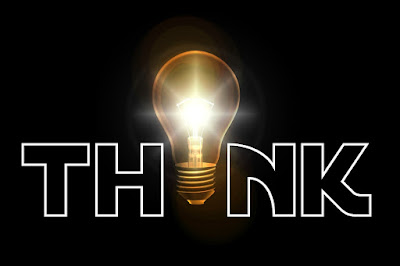## Saturday, 26 May 2018

### Electromagnetic Theory Multiple Choice Questions

Electronics and communication Multiple Choice Questions

1. The electric field intensity magnitude at a point (1,2,3) m, in Cartesian coordinates due to a charge(1/9) nC located at the origin is equal
a. 3.3 V/m
b. 0.33 V/m
c. 0.033 V/m
d. zero

2. The divergence theorem applies to?
a. static fields only
b. time-varying fields only
c. both static as well as time-varying fields
d. magnetic fields only

3. For a plane electromagnetic wave traveling in a linear, isotropic, homogeneous stationary medium?
a. E and H lie in the same plane
b. E * H = 0
c. E and H are mutually perpendicular
d. None of these

4. An electromagnetic wave propagates from a dielectric medium to the interface with free space. If the angle incidence is the critical angle of 30 degrees, the relative permittivity of the dielectric medium is?
a. 8
b. 4.5
c. 6.8
d. 4

5. When an electromagnetic wave traveling in one medium strike upon a second medium having a different conductivity the wave will be?
a. neither transmitted and partially reflected
b. totally reflected
c. partially transmitted and partially reflected
d. totally transmitted

6. In the perfect conductor, the incident and the reflected waves combine to produce?
a. linearly polarized
b. circularly polarized
c. elliptically polarized
d. horizontal polarized

7. The polarization of dielectric materials results in?
a. absorption of electrons
b. the release of high-velocity protons
c. creation of electric dipoles
d. production of eddy currents

8. The absorption of radio waves by the atmosphere depends on?
a. the polarization of atmosphere
b. the polarization of the waves
c. their frequency
d. their distance from the emitter

9. As an electromagnetic wave travels in free space the only one of the following can happen to them?
a. reflection
b. absorption
c. attenuation
d. refraction

10. When a current carrying conductor is brought into the magnetic field the force that moves the conductor depends upon which of the following?
a. The value of current
b. The weight of conductor
c. The direction of the conductor
d.  None of these

1.  b
2. c
3. c
4. d
5. c
6. c
7. c
8. c
9. c
10. a